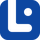# Articles related to "subordinating conjunctions"

### Although vs. Yet

'Although' and 'yet' can express the meaning 'but.' In this lesson, we will learn the similarities and differences between these two conjunctions.

### Subordinating Conjunctions

Subordinating conjunctions joins subordinate or dependent clauses to the main or independent clauses. To know all about these tricky grammatical words, click!

### Either vs. Whether

This is one of the easiest grammatical points in the English language. But in some ways, it can be a little tricky. Let's start.

As you know, clauses have subjects and verbs. Adverbial clauses are clauses that function as the adverb of the sentence.

### Since

'Since' has different functions in the English language. In this lesson, we will discover more details about this word and learn when to use it.

### Whether vs. Rather

'Whether' and 'rather' are confused by earners. In this lesson, we will learn their uses and differences.

### If

You may want to set out a few conditions. In this case, you can use the word 'if,' but you must know its rules and uses. In this lesson, we will learn more.

### Though

'Though' is commonly used in spoken English. It functions as both a conjunction and an adverb. In this lesson, we will discover more about it.

### Because

In this lesson, we want to explore the word 'because' since it is very common in sentences.

### Conjunctions of Place

The conjunction of place is a kind of subordinating conjunction talking about the whereabouts of something. In this lesson, we will learn all about them.

### While

'While' is a commonly used word that has many functions. In this lesson, we will discover all about it.

### Once vs. After

'Once' and 'after' are conjunctions of time. In this lesson, we will discover their differences and similarities.

### Compound-complex Sentences

A compound-complex sentence is comprised of at least two independent clauses and one or more dependent clauses. Let's get to know it in detail!

### Although

You may have noticed the word 'although' in many sentences. 'Although' mainly functions as a conjunction. In this lesson, we will discover more.

### For

'For' is a widely used preposition. However, that is not its only function. In this lesson, we will discuss all about this word.

### Once

'Once' in a while, you may encounter the word 'once.' In this lesson, we will discover more about this word and its uses.

### Conjunctions of Cause and Effect

Conjunctions of cause and effect are used to express the reason or the result of an action. In this lesson, we will learn all about them.

### Unless

'Unless' is commonly used by English language speakers. In this lesson, we will learn all about this word and when and how to use it.

### Why vs. Because

'Why' and 'because' are mainly confused when it comes to their usage and meaning. In this lesson, we will learn their uses and differences.

### Conjunctions of Time

Conjunctions of time connect two clauses while stating the time. In this lesson, we will learn all about them.

### Although vs. But

'Although' and 'but' are conjunctions that express contrast. In this lesson, we will learn more about them.

### Conjunctions

To connect two sentences to each other, we need some words to link them without breaking the structure of the sentences. Let us learn these linking words.

### For vs. Since

This time, we're gonna analyze two confusing grammatical prepositions. These two are widely used among native speakers.

### Neither vs. Nor

'Neither' and 'nor' are easily used by native speakers, but they are somehow hard for beginners. So, let us start learning about these two words.

### Complex Sentences

A complex sentence is a sentence that contains an independent clause and one or more dependent clauses. In this lesson, we will learn all about this type!

### Like

I'm sure you all have heard 'like' a lot, but are you familiar with all its functions? We'll learn all about it in this lesson.

### Before

'You have heard this before.' You may have noticed 'before' in such sentences. In this lesson, we will discover more about it.

### After

The word 'after' functions as a preposition, conjunction, adverb, and adjective. In this lesson, we will discover this word in detail.

### Conjunctions of Concession

Conjunctions are categorized into different groups. In this lesson, we will learn about conjunctions of concession.

### Because vs. So

'Because' and 'so' may confuse learners as they are both conjunctions. In this lesson, we will learn their differences.

### Until

'Until' is a word that is commonly used in the English language. In this lesson, we will learn more about it and its uses.

### Than

'I like the red one more than the blue one.' You have probably heard 'than' in sentences like these. In this lesson, we will uncover all about it.

### When vs. Where

'When' and 'where' are wh-words commonly used in the English language. In this lesson, we will learn more about them and their similarities and differnces.

### Then vs. Than

This time, we're gonna discuss the differences and similarities between these words. Well, what do you think? Wanna learn more?

### Conjunctions of Condition

As their name requires, conjunctions of condition are used to express events that need a special condition to happen.

### Whether

I'm pretty sure you've heard 'whether' before, haven't you? Well, in this lesson, we are gonna see what we can learn about this word.